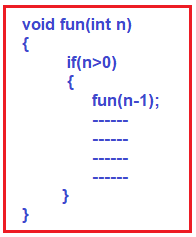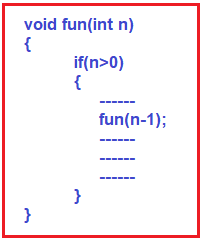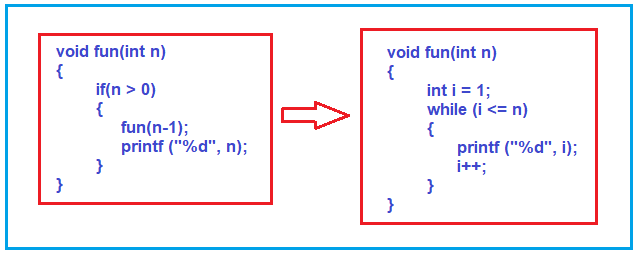## Head Recursion in C Language with Examples

##### Head Recursion in C Language:

Now let us understand Head Recursion. Following is the structure of head recursion. If there is a function that is calling itself, then it is a recursive function. As you can see in the below image, the function fun calling itself, so it is a recursive function. Then if you further notice the first statement inside the function is the recursive call. That means, what all the processing it has to do, it is doing at returning time i.e. after the recursive call. There is no statement that is no operation before the function call.Note: If there is something before the recursive call then it is not a head recursion. If something is there before the function call, it is just a recursion. We don’t have to give any special name for it. The following is not head recursion.##### What do you mean by head recursion?

Head Recursion means the function doesn’t have to process or perform any operation at the time of calling; it has to do everything only at the time of returning. If all the processing or operations are done at the returning time then such recursive functions are called head recursion.

##### Example: Head Recursion in C Language

The following is an example of Head Recursion and we have already seen such types of examples in our previous articles. As you can see in the below example, within the if block the first statement is the recursive function call. Before the recursive call, there is no statement present which means it is not performing any operation at the calling time. Further, if you notice, after the recursive function call, the printf statement is there which is going to be executed at returning time. And hence this is an example of Head Recursion.

```#include <stdio.h>
void fun(int n)
{
if(n > 0)
{
fun(n-1);
printf ("%d", n);
}
}

int main()
{
fun(3);
return 0;
}
```

Output: 123

##### Comparing Head Recursion with Loop in C Language:

Now we will compare head recursion with loop. Before comparing the first question is, can we convert head recursion into a loop? Yes, we can. For this, we need to write some logic. Let us see, how to convert a head recursion to a loop. Please have a look at the following image.The following code shows the complete example using a loop.

```#include <stdio.h>
void fun(int n)
{
int i = 1;
while (i <= n)
{
printf ("%d", i);
i++;
}
}

int main()
{
fun (3);
return 0;
}
```

Output: 123

Note: The point that you need to remember is if a recursive function does some operation at returning then it is not easy to convert that recursive function to a loop. We need to convert by writing some logic.

Time Complexity: The time complexity of Head Recursion is O(n).

In the next article, I am going to discuss Tree Recursion in C Language with Examples. Here, in this article, I try to explain Head Recursion in C with Examples and I hope you enjoy this Head Recursion in C article.